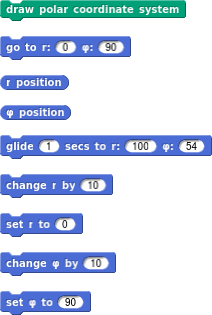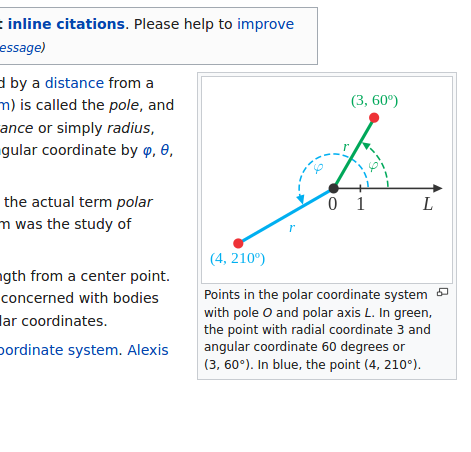# Polar coordinate system

See inside!

​ ​ ​ New Blocks:Nice

I love that green curve! It took me a while to figure out what it was doing.

Is there a reason you used phi instead of theta for the angle?

I chose phi, because it's one of my favorite Greek letters and Wikipedia uses it, too.I think theta is normally used for 3-dimensional stuff, phi for 2 dimensions.

How can I attach projects to forum posts?

Go to the project page, click the Embed button, copy the HTML, and paste it in a forum post.

Huh. It's been a long time since I was in high school, but I could have sworn it was the other way around. Theta always comes first, e.g., sin(θ+φ)= [let's see if I can do this from memory...]2(sin θ cos φ + cos θ sin φ). Oh well, I was close. The coefficient 2 is wrong; that's part of the sin(θ)+sin(φ) formula. Also Wolfram Alpha doesn't like using φ for an angle because it interprets φ as the golden ratio. :~/ I had to change it to sin(a+b) to get the answer.

Correct, theta is the primary symbol for representing an angle, and phi comes next (in polar coordinates, phi is the second angle)